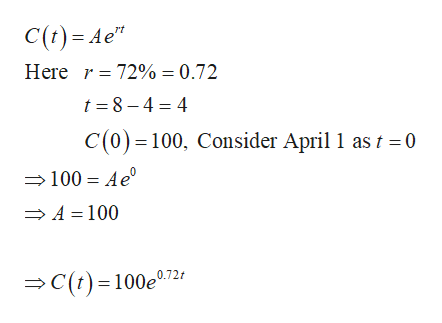# In the first six months of the 2014 Ebola outbreak, the total number of reported cases was increasing at a continuous rate of about 72% per month. There were about 100 cases as of April 1, 2014. Find an exponential model in the form C(t)=Ae^rt  for the number of cases "t" months after April 1, 2014, and use it to predict the number of cases as of August 1, 2014. (The actual number of reported cases was 1603 .)

Question
43 views

In the first six months of the 2014 Ebola outbreak, the total number of reported cases was increasing at a continuous rate of about 72% per month. There were about 100 cases as of April 1, 2014. Find an exponential model in the form C(t)=Ae^rt  for the number of cases "t" months after April 1, 2014, and use it to predict the number of cases as of August 1, 2014. (The actual number of reported cases was 1603 .)

check_circle

Step 1

There were 100 cases as of April 2014 and it increases continuously at the rate of 72% per...help_outlineImage TranscriptioncloseC(t) = Ae" Here r = 72% = 0.72 t = 8 – 4 = 4 C(0) = 100, Consider April 1 as t = 0 =100 = Ae' → A = 100 >C(t)=100e0.724 fullscreen

### Want to see the full answer?

See Solution

#### Want to see this answer and more?

Solutions are written by subject experts who are available 24/7. Questions are typically answered within 1 hour.*

See Solution
*Response times may vary by subject and question.
Tagged in

### Math